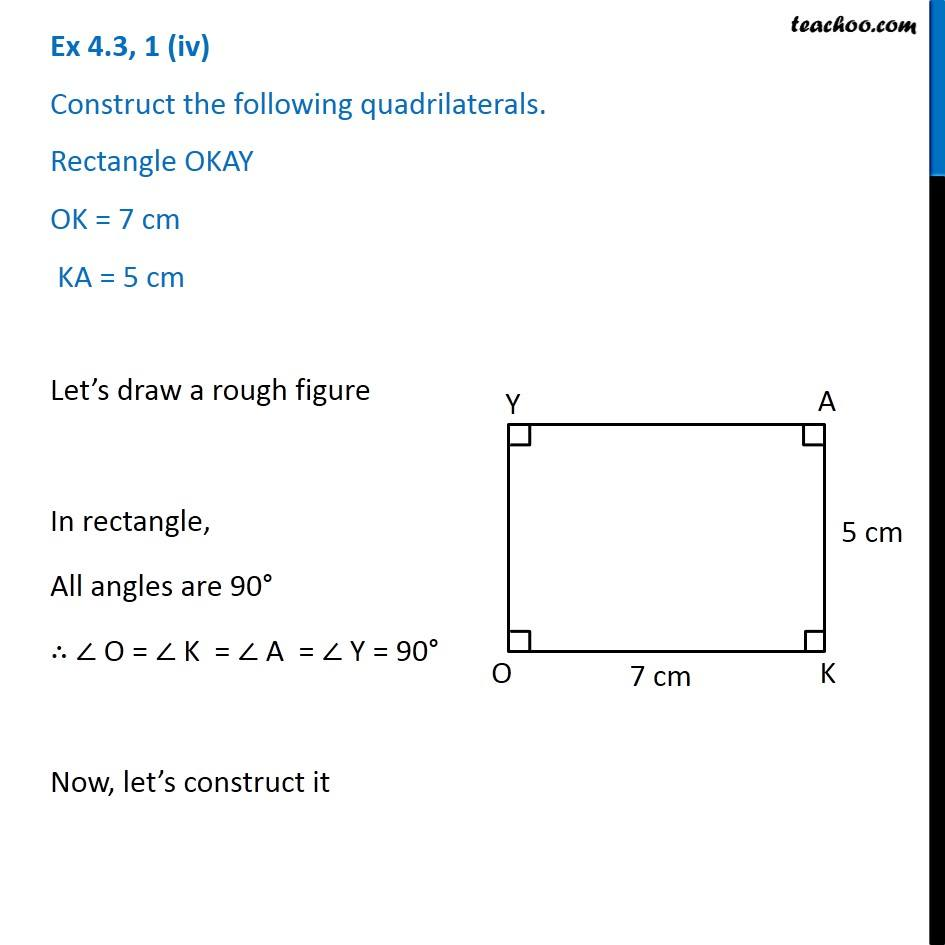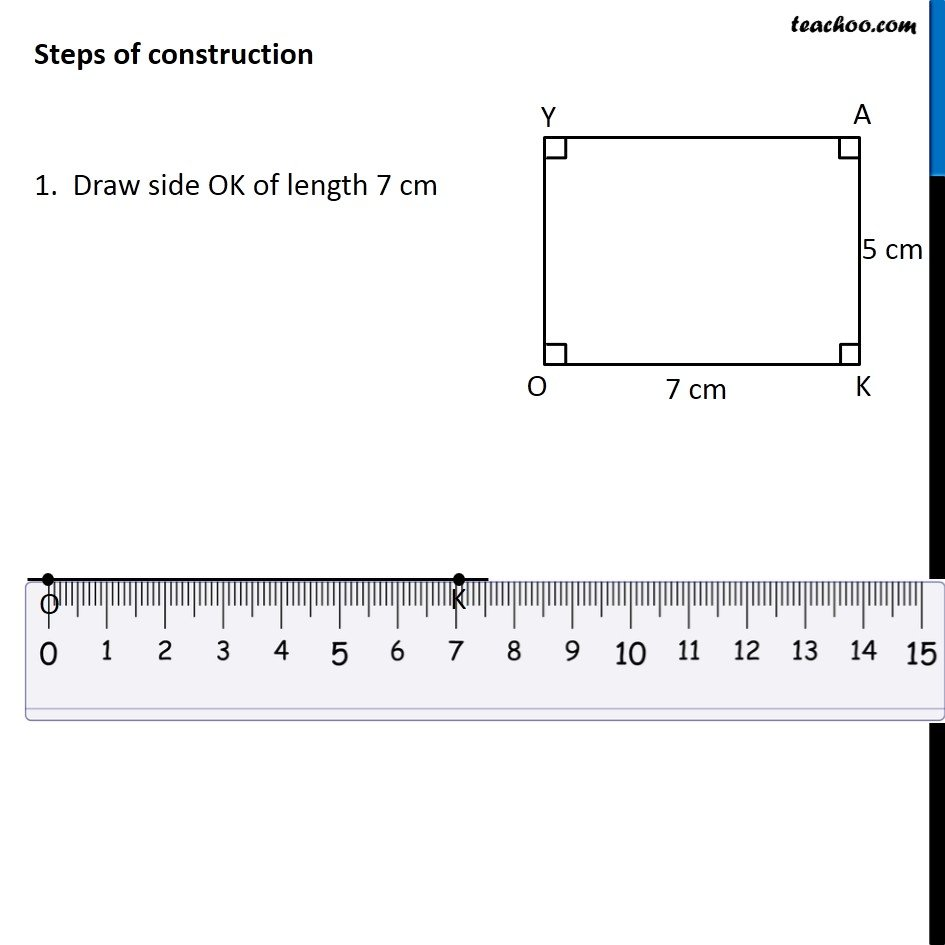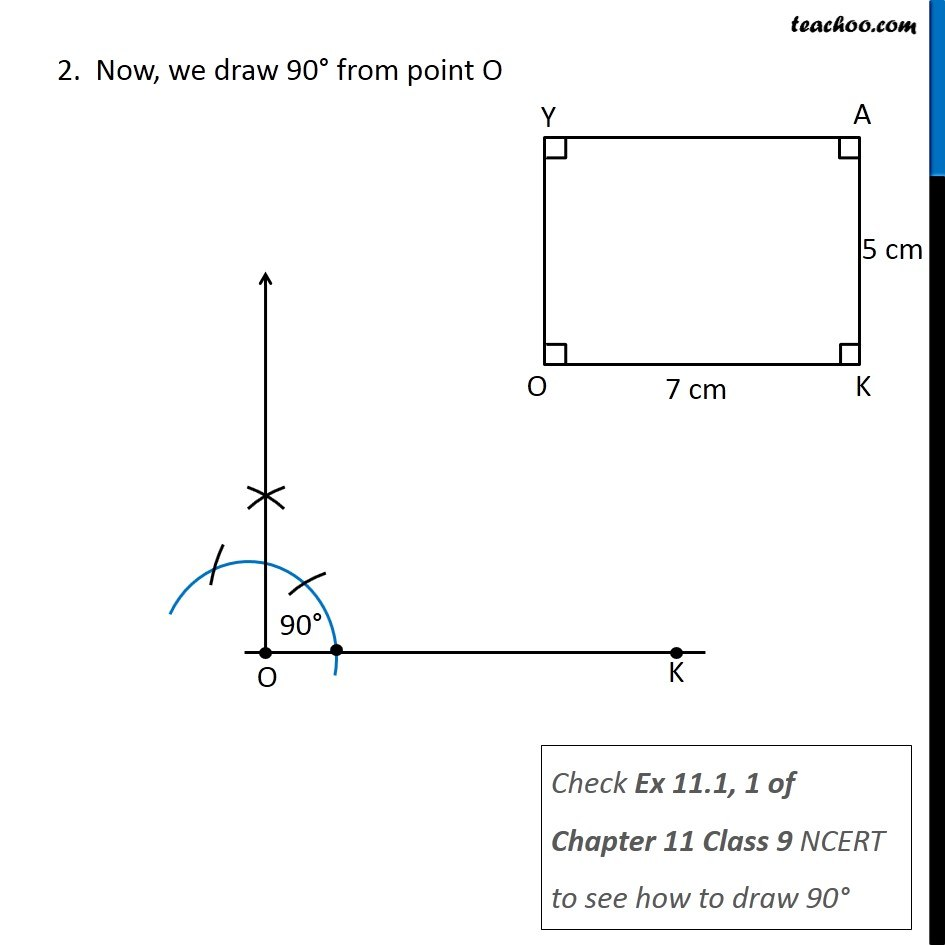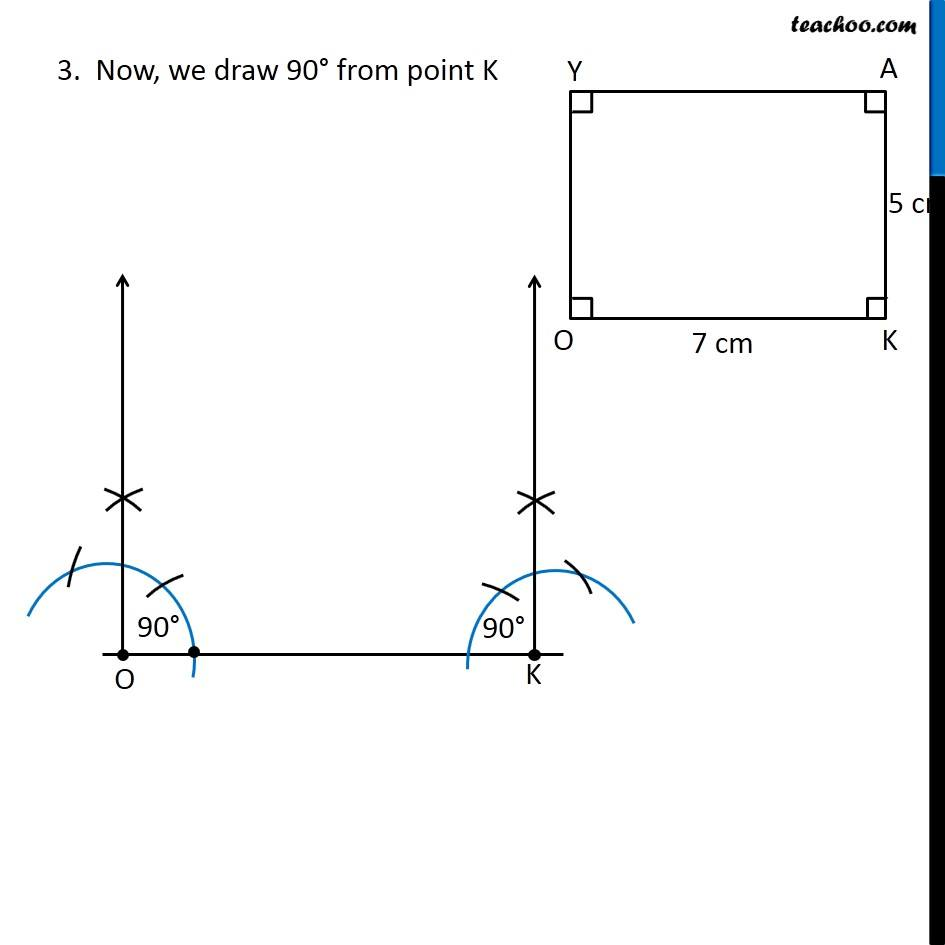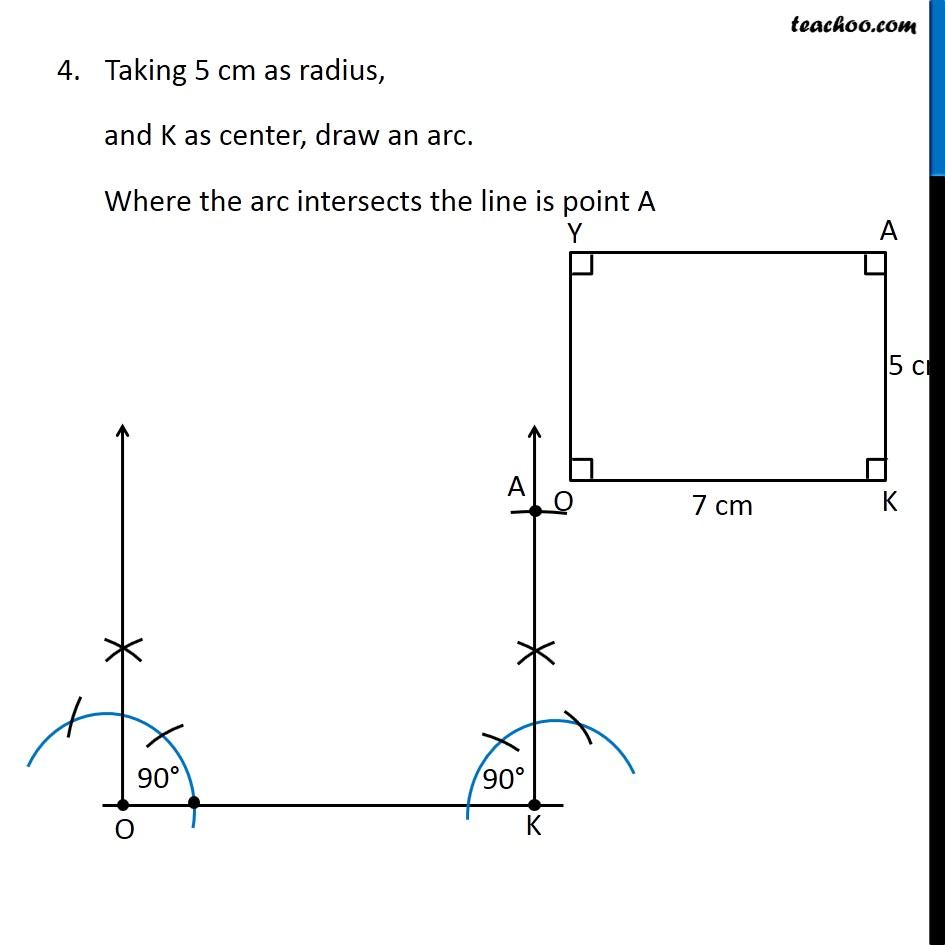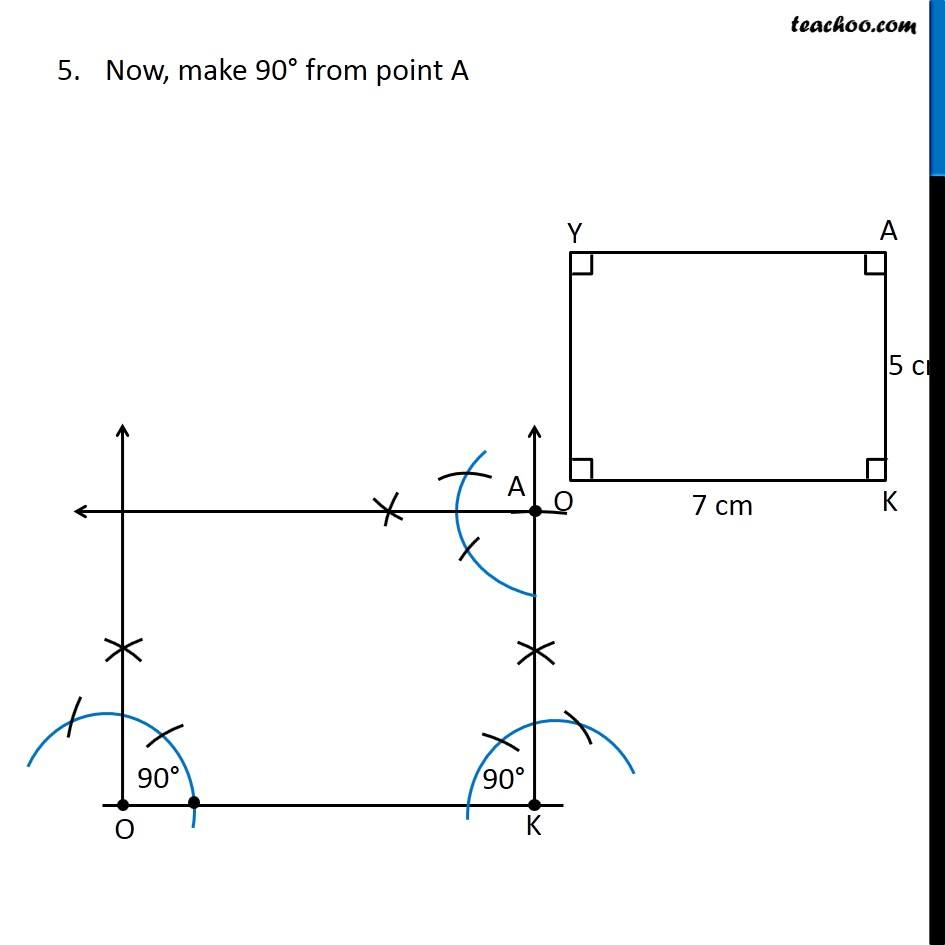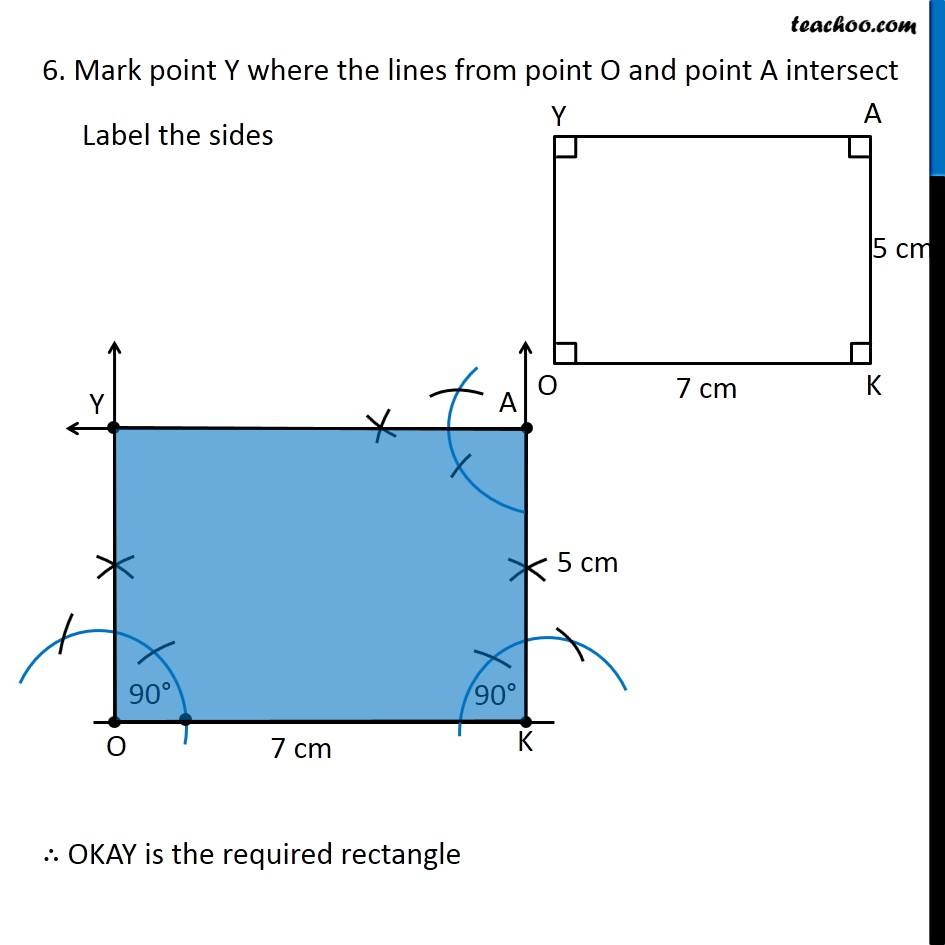Learn in your speed, with individual attention - Teachoo Maths 1-on-1 Class

### Transcript

Question 1 (iv) Construct the following quadrilaterals. Rectangle OKAY OK = 7 cm KA = 5 cm Let’s draw a rough figure In rectangle, All angles are 90° ∴ ∠ O = ∠ K = ∠ A = ∠ Y = 90° Now, let’s construct it Steps of construction 1. Draw side OK of length 7 cm 2. Now, we draw 90° from point O Check Ex 11.1, 1 of Chapter 11 Class 9 NCERT to see how to draw 90° 3. Now, we draw 90° from point K Taking 5 cm as radius, and K as center, draw an arc. Where the arc intersects the line is point A Now, make 90° from point A 6. Mark point Y where the lines from point O and point A intersect Label the sides ∴ OKAY is the required rectangle